# Surface Area & Volume Of Spheres

in STEMGeeks2 months ago

Hi there. In this short mathematics post, I will cover the surface area and volume of a sphere.

Math text rendered in LaTeX with QuickLatex.com.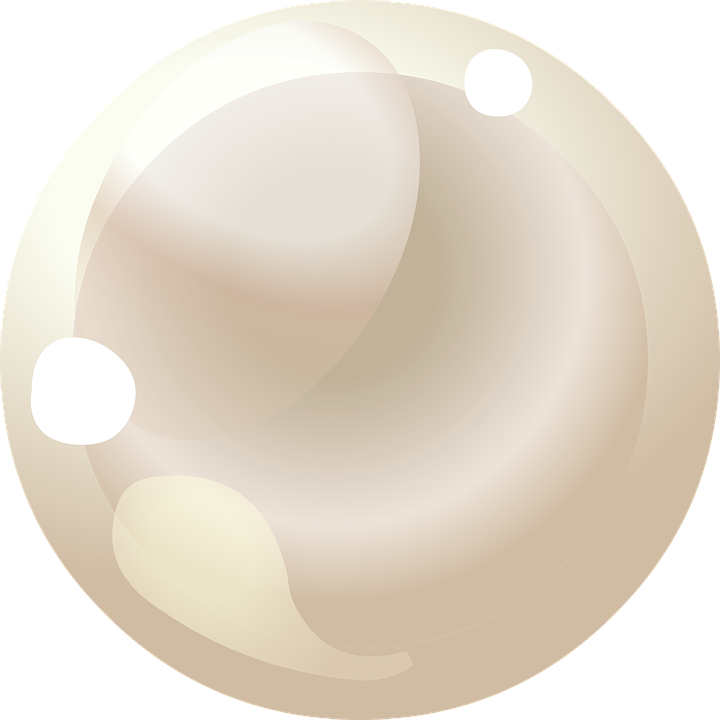Pixabay Image Source

## Topics

• Basic Sphere Properties
• Surface Area Of A Sphere
• Volume Of A Sphere
• Examples

## Basic Sphere Properties

The sphere is a three dimensional solid figure which has no corners, no edges and is perfectly symmetrical. All points outside the sphere's surface are of equal distance to the centre of the sphere. This equal distance from the centre to a point on the sphere's surface is the radius.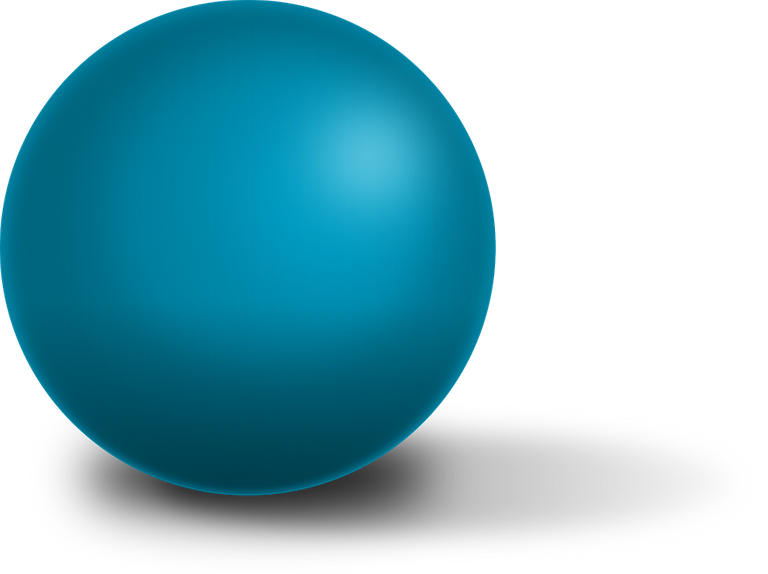Pixabay Image Source

## Surface Area Of A Sphere

Surface area refers to the area of a solid's outside surface. For the sphere, the surface area is the outside of the solid. The formula for the surface area of the sphere is given by: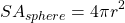## Volume Of A Sphere

Volume refers to the amount of space inside a three dimensional object. A sphere's volume is given by the formula: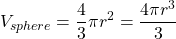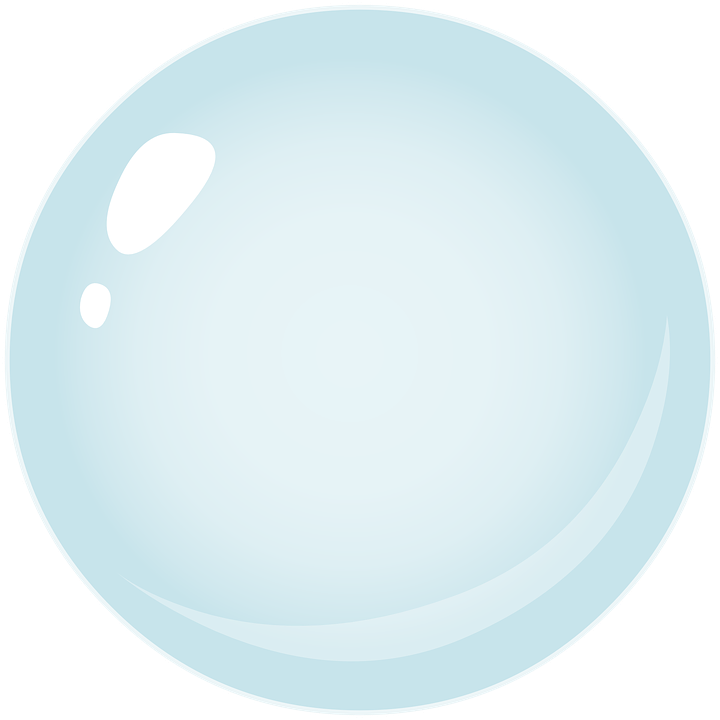Pixabay Image Source

## Examples

Example One

What is the surface area of a sphere with a radius of 7 centimetres?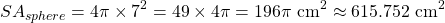Example Two

Determine the surface area of a sphere with a diameter of 10 centimetres.

Avoid the common mistake of mixing up the diameter and the radius. As the radius is needed take half of the diameter to obtain 5 centimetres and then use this for the surface area formula.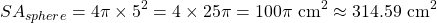Example Three

Given a radius of 2 meters for a sphere, what is this sphere's volume?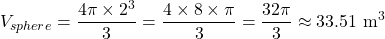Example Four

Find the volume of a sphere with a diameter of 20 centimetres.

Taking half of 20 centimetres gives a radius of 10 centimetres.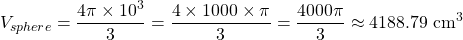Using the formula for the surface area and volume of there sphere is not too difficult. I have not covered finding the radius given the surface area or volume of the sphere. That would involve taking the square root or the cube root of a number which is another topic.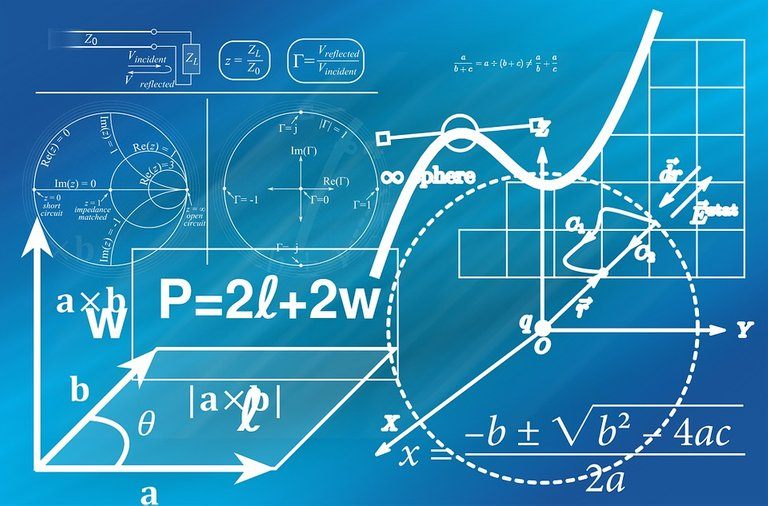Pixabay Image Source

Thank you for reading.

Posted with STEMGeeks